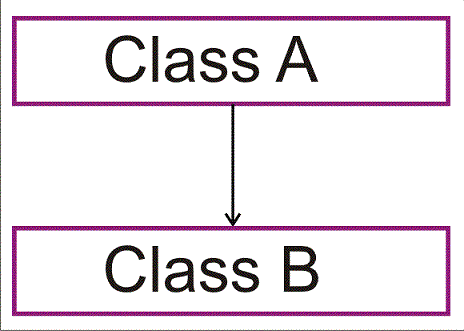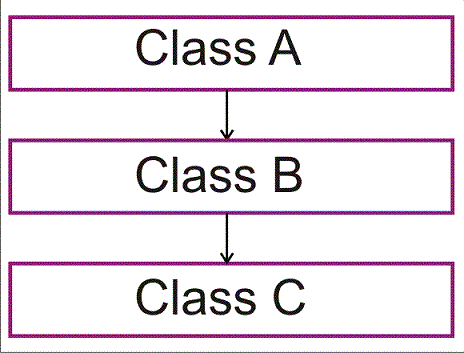# C++ language

Types of Inheritance (Single, Multiple)
 Previous Home Next

### Types of Inheritance:

• Single Inheritance
• Multiple Inheritance
• Hierarchal Inheritance
• Multilevel Inheritance
• Hybrid Inheritance

Single Inheritance:

One derived class inherit the public members of base class. In single inheritance there is single base class and also single derived class .Derived class can access only public member of base class and can access the public member through derived class objects.Base class

```#include<iostream>
class Cals 		  // Base class
{
private:
float number1 ; // private member and data can't inherited
public:
float number2;    // The public member and data are inheritable
void getdata()	 // The public member and data are inheritable
{
cout <<
"\n Enter the number1 of the Cals : ";
cin >> number1 ;
cout <<
"\n Enter the number2of the Cals : ";
cin >> number2;
}
float first_number()
// The public member and data are inheritable
{ return number1 ; }
}; 				         // End of the class definition
```

Derived class

```class Calculate :
public Cals 		 //Derived class
{
private:
float result1 ,result2,result3,result4;
public:
void sum(void)
{
result1 = first_number() + number2;
//number1 can't access directly because its a private member of base class
}
void substract(void)
{
result2 = first_number() - number2;
//number1 can't access directly because its a private member of base class
}
void multi(void)
{
result3 = first_number() * number2;
//number1 can't access directly because its a private member of base class
}
void div(void)
{
result4 = first_number() / number2;
//number1 can't access directly because its a private member of base class
}

void Display(void)
{
cout <<
"\n number1 = " << first_number() ;
//number1 can't access directly because its a private member of base class
cout <<
"\n number2= " << number2;
cout <<
"\n sum is = " << result<< "\n substraction is = "
<<result2<<
"\n multiplication is = "<<result3<<
"\n division  is = "<<result4;
}
};			     // End of the derived class definition
void main(void)
{
Calculate cal ;
cal.first_number():
// The public member and data so its access through the object of derived class.
cal.sum( );
cal.substract( );
cal.multi( );
cal.div( );
cal.Display( );
}
```

Multiple Inheritance:

In multiple inheritance there are two are more base class is there. When a derived class inherit the member function of two or more classes .Multiple inheritance allow to combine the feature of more then two classes with in a single derived class.Derived class:

``` class derive: public
base,public base1,
public base2
{
.....
.....
.....       //member of derive class
};
```
```#include<iostream>
using namespace inherit;

class base1	  //base class1
{
protected:
int a;
public:
void get_data(int);
};

class base2		 //base class2
{
protected:
int b:
public:
void get_data1(int);
};

class derive:
public base1,public base2
//derived class inherit the feature and attribute of base1 class, base2 class
{
public:
void display(void);
};
void base1::get_data(int i)
{
a=i;
}
void base2::get_data1(int j)
{
b=j;
}
void derive::display(void)
{
cout<<
"value of base1 class variable is="<<a<<
"\n";
cout<<
"value of base2 class variable is="<<b<<
"\n";
cout<<
"result of a*b="<<a*b<<
"\n";
}
int main()
{
derive der;
der.get_data(200);
der.get_data1(300);
der.display();
return 0;
}
```

OUTPUT:

```value of base1 class variable is=200
value of base2 class variable is=300
result of a*b=60000
```
 Previous Home Next